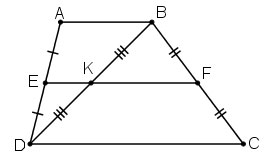# The formula for the median of a trapezoid

What is the median of a trapezoid, how is the formula to calculate the median of a trapezoid? Please refer to the article below for the answer.

## What is the median of a trapezoid?

The median of a trapezoid is the line segment joining the midpoints of the two sides of the trapezoid.

The theorem about the midsegment of a trapezoid

• The line passing through the midpoint of one side of the trapezoid and parallel to the two bases passes through the midpoint of the second side.
• The median of a trapezoid is parallel to the two bases and is half the sum of the two bases.

## The formula for the median of a trapezoid

The median of a trapezoid is half the sum of the two bases.

Trapezoid ABCD(AB//CD) has E, F being the midpoints of the two sides AD, BC, respectively.

Thus, EF is the median of trapezoid ABCD.

Then: AB//CD//EF andFrom this formula for calculating the average line of a trapezoid, you can combine it with the formula for calculating the area of ​​a trapezoid, the formula for calculating the height of the trapezoid, the formula for calculating the perimeter of the trapezoid to solve problems on shapes. ladder.

## Exercises on calculating the median of a trapezoid

Lesson 1: (Lesson 25, page 80, 8th grade math textbook, volume 1)

Trapezoid ABCD has bases AB and CD. Let E, F, K be the midpoints of AD, BC, BD, respectively. Prove that the three points E, K, F are collinear.Prize:

To prove that the 3 points E, F, K are collinear, we can prove that 2 of the 3 segments EK, FK, EF together // with AB and CD (according to the Euclidean axiom) through the property of the midline of the triangle and trapezoid.

Consider trapezoid ABCD, there are:

E is mid point of side AD (gt)

F is mid point of side BC (gt)

EF is the median of trapezoid ABCD (according to theorem 3)

EF // AB // CD (by theorem 4) (1)

Considering △ABD△ABD, there are:

E is mid point of AD (gt)

K is mid point of BD (gt)

EK is the median of triangle ABD (according to theorem 1)

⇒ EK // AB (according to theorem 2) (2)

From (1), (2) ⇒ E, F, K are collinear (According to Euclidean axiom).

Lesson 2: An isosceles trapezoid has sides of 2.5 cm and a median of 3 cm. Calculate the circumference of
that trapezoid.

Prize:

The sum of the two bottom sides of a trapezoid is: 3 x 2 = 6 (cm)

Perimeter of a trapezoid is: 6 + 2.5 + 2.5 = 11 (cm)